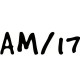Class 10 Maths Notes

# CBSE Class 10 Maths Chapter 8 Introduction to TrigonometryWritten by

### Introduction to TrigonometryIntroduction to Trigonometry

### Trigonometry

The word ‘trigonometry’ is derived from the Greek words ‘tri’ (meaning three), ‘gon’ (meaning sides) and ‘metron’ (meaning measure). In fact, trigonometry is the study of relationships between the sides and angles of a triangle.Application of Trigonometry
1. Find height of tall building or tower without actually measuring it
2. Find the height at which an object , airplane or balloon is flying
3. Find the distance of a vehicle or ship from a tower.
4. Used extensively in the field of construction.

### Trigonometric RatiosUsing this logic ,In right ΔABC

sine of ∠ A = P/H = BC/AC

cosine of ∠ A = B/H  = AB/AC

tangent of ∠ A = P/B = BC/AB
cosecant of ∠ A = 1/ sine of A = AC/BC
secant of ∠ A = 1/cosine of A  = AC/AB
cotangent of ∠ A = 1/tangent of A = AB/BC
The value of sin A or cos A never exceeds 1, whereas the value of sec A or cosec A is always greater than or equal to 1.
Trigonometric ratios of an acute angle in a right triangle express the relationship between the angle and the length of its sides.
The values of the trigonometric ratios of an angle do not vary with the lengths of the sides of the  triangle, if the angle remains the same.
Greek letter θ (theta) is also used to denote an angle.
Numerical: In Δ ABC, right-angled at B, AB = 24 cm, BC = 7 cm. Determine : sin A, cos A ,sin C, cos C.Solution:
We can find the third side, hypotenuse AC = √ AB+ BC2 = √242 + 72 = 25
Sin A = P/H = BC/AC  = 7/25
Cos A = B/H  = AB/AC = 24/25
Sin C = P/H = AB/AC=24/25
Cos C = B/H  =BC/AC = 7/25

### Trigonometric Ratios of Some Specific Angles

Let’s see the values of trigonometric ratios for angles 0°, 30°, 45°, 60° and 90°.From the table above you can observe that as ∠ A increases from 0° to 90°, sin A increases from 0 to 1 and cos A decreases from 1 to 0.
Numerical: In Δ ABC, right-angled at B, AB = 5 cm and ∠ ACB = 30°. Determine the lengths of the sides BC and AC.Solution:

Tan C =P/B  =AB/BC

Or Tan 300 = 5cm/BC

1/√3 = 5cm /BC    (using table, tan 300 =1/√3).

Or BC = 5√3 cm.

Now we can find the third side by Pythagoras Theorem. We can also find third side using trigonometric identities.

Sin C = P/H = AB/AC

Or Sin 300 = 5cm/AC

Or     1/2  = 5cm /AC  (using table, Sin 300 =1/2).

Or AC = 10 cm.

### Trigonometric Ratios of Complimentary Angles

Two angles are said to be complementary if their sum equals 900sin (90° – A) = cos A,
cos (90° – A) = sin A,
tan (90° – A) = cot A,
cot (90° – A) = tan A,
sec (90° – A) = cosec A,
cosec (90° – A) = sec A,
Memory tip:
sin ècos… and  cos è sin… !!!!  tan è cot …. And cot è tan.
Numerical: Evaluate tan 65°/ cot 25°
cot A = tan (90° – A)
So, cot 25° = tan (90° – 25°) = tan 65°
Thus tan 65°/ cot 25°  = tan 65°/ tan 65°  = 1

### Trigonometric Identities

sin2 A + cos2 A = 1,

secA – tan2 A = 1 for 0° ≤ A < 90°,

cosec2 A = 1 + cot2 A for 0° < A ≤ 90°.

Numerical: Express the ratios cos A, tan A and sec A in terms of sin A.

Solution:     cos2 A + sin2 A = 1,

Or  cos2 A = 1 – sin2 A, i.e., cos A = ±√ 1 − sin2 A

or  cos A = √1 − sinA      (Ignoring the negative value , since sin A is positive, Cos A will also be positive.)

tan A = sin A/cos A   = sin A/√1 − sinA

sec A = 1/cosA  = 1/√1 − sinA

### MATHS Revision Notes

Chapter:01  Real Numbers System
Chapter:02  Polynomials

Chapter:05  Arithemetic Progressions
Chapter:06  Triangles
Chapter:07  Coordinate Geometry
Chapter:08  Introduction to Trignometry
Chapter:09  Some Application Of Trignometry
Chapter:10  Circles
Chapter:11  Constructions
Chapter:12  Area Related to Cirles
Chapter:13  Surface Area Volume
Chapter:14  Stastistics
Chapter:15  Probability

Science Revision Notes

English Revision Notes

Economics Revision Notes